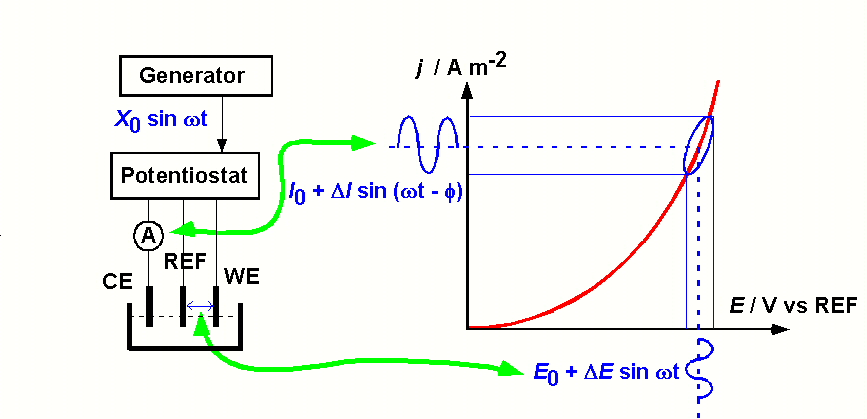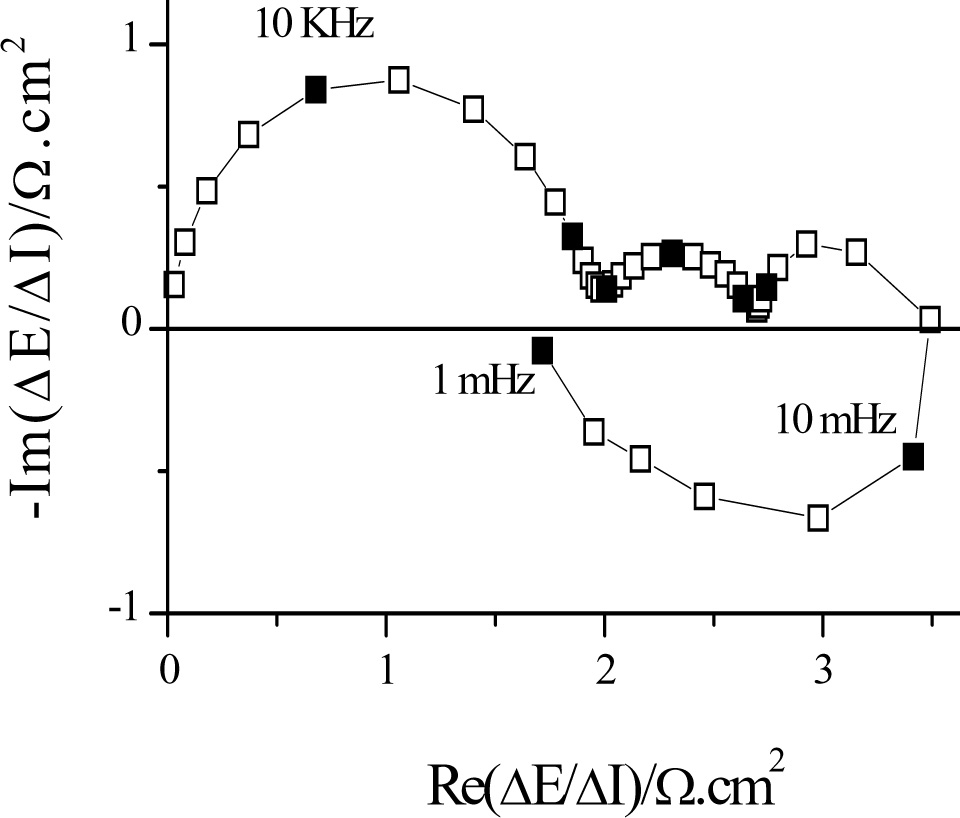# Electrochemical Impedance or EIS

In addition to stationary techniques (generally current-voltage relationships) that allow the simplest electrochemical processes to be studied, non-stationary techniques are needed to analyze more complex electrochemical systems. The use of the latter is based on principles similar to those that justify the use of relaxation methods in chemical kinetics at equilibrium. A disturbance in the electrochemical system displaces the reactions from their stationary state. As the various elementary processes evolve at different rates, the system response can be analyzed to dissect the overall electrochemical process.

Among non-stationary techniques, impedance techniques are increasingly used in electrochemistry both for academic studies and industrial applications to characterize electrode processes. This technique is based on the analysis of the current (potential) response to a small, often sinusoidal, disturbance of the potential (current) (Figure 1). The measurement is carried out at constant polarization potential, as soon as the interface has reached a steady state, by varying the analysis frequency over a wide frequency range (often from 50 kHz to 0.001 Hz). This measurement is then repeated throughout the current-voltage curve in the potential range studied. This technique is often found in the Anglo-Saxon literature under the name of "Electrochemical Impedance Spectroscopy" (EIS).

 Figure 1: Principle of electrochemical impédance measurementFigure 2: Impedance measured during copper deposition in a sulfate/sulfuric medium in the presence of additives

The electrochemical impedance contains information about the processes that take place at the interface (electrochemical and chemical reactions, diffusion,...) and its structure. Plotted in the complex plane (Re[Z(f)],-Im[Z(f)), the high frequency limit generally gives the electrolyte resistance and the low frequency limit gives the polarization resistance (inverse to the slope of the current-voltage curve) (Figure 2). In high frequency, there is a capacitive loop linked to the parallel connection of the load transfer resistance and the double layer capacitance. In lower frequency, we can find capacitive or inductive semicircles which represent the relaxations of the reaction intermediates, capacitive diffusion loops characterized by parts at 45° with respect to the real axis, negative resistances in the case of passivation...

The experimental results can be interpreted in two ways. On the one hand, we can look for an equivalent circuit with the same impedance. On the other hand, we can look for a model involving kinetic equations describing reactions and material transport and after linearization calculate a theoretical impedance that can be compared to experimental data.

Each year, LISE laboratory organizes a meeting entitled "Forum on Electrochemical Impedances" where academic and industrial users of this technique meet. In addition, training is given each year at LISE as part of the Continuing Education program at Sorbonne University, where particular emphasis is placed on modelling and instruction in instrumentation.

Reference : C. Gabrielli, Identification of electrochemical processes by frequency response analysis, Ed. Solartron, 120 pages. (format pdf)